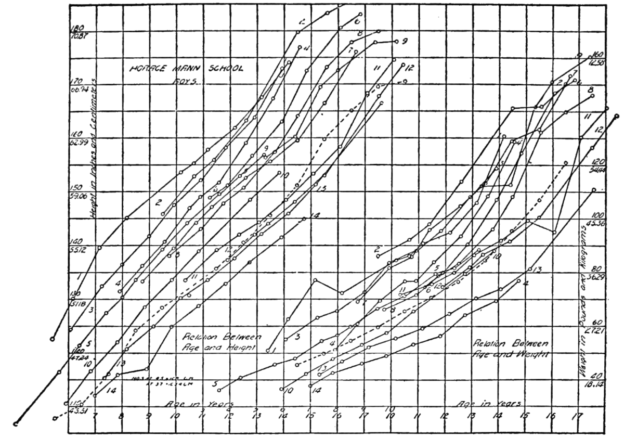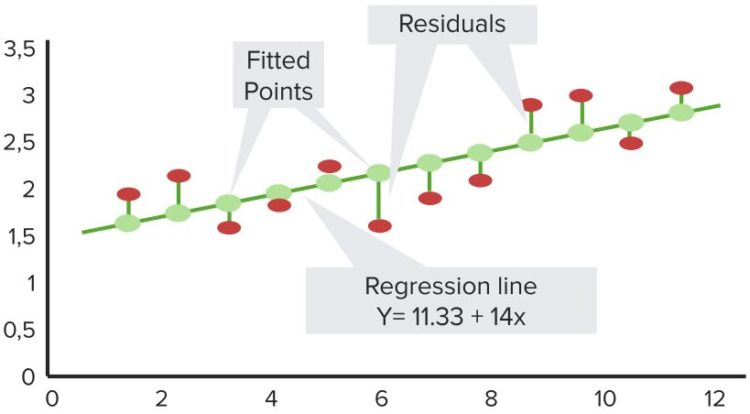Are you more of a visual learner? Check out our online video lectures and start your statistics course now for free!Image: “Table of height and weight for boys.” by Unknown – Popular Science Monthly Volume 85. License: Public Domain

## The Linear Model (Describing Relationship with Lines)

It is the most widely used technique of data analysis and measurement in statistics. This model is aimed at explaining the relationship between two quantitative variables with a line. Of these two quantitative variables, one variable is of independent nature, whereas the other is dependent on nature. Normally, in a linear model, “Y” is considered as the dependent variable and “X” as the independent variable. The equation of a straight line or linear model comprises of the following factors:

Y = Dependent variable

X = Independent variable

t = Time period

b = Coefficient of variable

a = Slope of intercept

### Assumptions of linear regression

The assumptions that the relationship between the dependent and independent variables should be linear are based on the following considered facts:

• The linear relationships between variables are non-trivial relationships which are imaginary.
• The true relationship between variables is often and at least linear over the range of values.
• Despite the fact that the relationship between variables is not linear, we can linearize it accordingly by the transformation of variables.

## Residuals

This model of linear regression helps in measuring imperfections in data. In the case of the linear model, the straight line may deviate from data to the line. These deviations create imperfections in linear regression analysis.

In order to find out the required linear relationship between variables, we predict a value and observe it later using linear regression formulas. The observations which are observed using linear models are denoted by “y”. The values which are predicted before the data analysis process are denoted by “ŷ”. The difference between observed values y and predicted values ŷ are termed as residuals.“Residuals” Image created by Lecturio

### The regression line

This line helps in measuring line which fits best with available data. Larger a residual, it shows that a line poorly fits the available data. The regression line creates a line which fits best with given data with small residual values. The sum of positive and negative residuals is always zero.

The regression line helps in finding out the line which minimizes the sum of all residuals. Eventually, positive residuals cancel the effect of negative residuals and the ultimate effect of residual is zero.

A regression line is the one which minimizes the sum of the squares of all residuals of available data. When we find out the line which plays the role of the regression line, it is considered a line of best fit or least square line.

The formula of the regression line is given as follows:

Y’ = bx + a

### Calculating the Y-intercept

The y-intercept is the value which is measured when the regression line hits Y-axis. It tells about the expected value of y or dependent variable when the value of independent value x = 0. It is simply the value which is measured at the point regression line intersects Y-axis.

The formula of Y-intercept is the same as linear regression i.e. y = bx + a.

Suppose the value of b = 4, if x value = -1 and y value = -6. Putting the values in Y-intercept formula, the value of b comes out:

(–6) = (4) (–1) + a

–6 = –4 + a

–2 = a (y-intercept)

### Using the slope to find the intercept

The slope of the data can be measured by analyzing data which is the steepest. The slope and intercept of an equation indicate the relationship between the independent and dependent variable. It finds out the average rate of change of variable. In case the magnitude has a huge slope, the regression line becomes steeper which indicates the higher rate of change.

## Extrapolation

This is the process of making predictions outside the values available of a given data. In case a prediction about the response variable is huge outside the range of given data; it becomes riskier and difficult to predict the continuation of a linear relationship between independent and dependent variables.

Extrapolation uses the regression equation to make a prediction about the correspondence of explanatory and response variables. Response variables are dependent (Y) whereas explanatory variables are independent variables (X) in a data set.

Extrapolation involves regressions equation for prediction outside the data range despite the fact that it does not indicate what is happening outside the range of given data. It should be avoided to make predictions outside the range of given data.

## Using Regression as a Crystal Ball

A regression line helps in predicting the value of the dependent variable “Y” by observing the impact of the independent variable “X”. In moderate correlation between two variables through scatterplot and correlation coefficient, indications are there that some kind of linear relationship exists.

While using regression as a crystal ball, it is relevant to find out which variable will play the role of a dependent value and which one will be taken as X or dependent variable. In order to find the best fitting lines for valuable predictions, the choice of X and Y makes a difference. In order to make a correct prediction regarding Y, the following conditions are required to be met:

• The scatterplot of given data should create a linear pattern.
• The correlations coefficient i.e. r should be moderate or lying between +0.50 or -0.50.

### Revisiting residuals

In order to find the appropriateness of a linear regression model for making a prediction of response and explanatory variables, it is necessary to look at the distribution of residuals. If residuals show a normal distribution, the prediction about the response variable becomes easy and clear.

In case the residuals are not normally distributed, it indicates a deviation from linearity. Values are scattered and deviate from a straight line showing that the line is not the best fit.

## Variation in Regression Model

The variation accounted for the relationship between the response variable (Y) and explanatory variable (X) can be measured by using R-squared quantity denoted by R2. R-squared quantity indicates the percentage of variation between the values of X and Y. The simplest way to calculate R-squared quantity is by squaring the correlation.

### R2 (rule of thumb)

There is no rule of thumb for a good value of R2 in the dataset. It varies from data to data. Scientific experimental data normally has R2 between 80 and 90%. Observations have shown lower R2 value is useful if lies between 30 and 50%.

## Appropriateness of Linear Regression Model

In order to get effective results by the use of a linear regression model, the following four conditions should be met:

### 1. Quantitative variable condition

Both dependent and independent variables of a data set, neither of the variables should be categorical. A categorical variable is the one which can take a fixed number of limited value, further assigning the other variable a specific category based on its quantitative property. In case any of the variables are categorical, the linear regression model should be stopped immediately.

### 2. Straight on scatterplot condition

A scatterplot helps in finding out whether the regression line fits best in a straight line or not. If the scatterplot shows the dispersion of residuals, it should be stopped.

### 3. Outlier condition

A scatterplot should be used to identify outliers. In case outliers are identified, the linear regression model does not work best with such data. Outliers are observations which show larger value than predictor values, response or dependent variable i.e. Y in this case.

### 4. Consistency of explanatory variable with straight line

The straight line of linear regression graph should be aligned with values of explanatory or independent variables (X).

In case any of the above given four conditions is missing, the data set is not suitable for linear regression model.

## Checking Assumptions of Regression Model

• The fitted values should be checked by drawing a plot of the residuals.
• In case of any bend in the plot, there is an indication of non-alignment of the explanatory variable in a straight line.
• Find any outlier it may occur after completion of regression calculation and at the stage of drawing scatterplot.
• Checking for any vertical spread of values from one part of the plot to the other.
• Ideally random scatter “0” is considered the best fit of regression line on the graph which is practically hard to achieve.

### Residuals vs. fitted values plot results

In case the following observations are noted from the residual plot, it indicates the violation of straight-line condition which is a worrisome factor:

• The plot has shown a huge and steep bend of values.
• The thickness of the plot increased when fitted value lies 0.50 to 0.60.

## Common Regression Mistakes

Normally, the following mistakes are practiced by researchers while using the linear regression model which should be avoided to get the desired results.

• The linear regression model should not be used for the non-linear relationship between two or more variables.
• Outliers are ignored which further create a violation of the straight-line relationship between variables.
• It is considered that independent variable X causes dependent variable Y to occur which is incorrect. X only influences Y due to the strong linear relationship between these two.
• The choice of X and Y is not made at the initial stage of the linear regression process.
• It may be possible that the regression line is used to predict X from Y.
Learn. Apply. Retain.
Your path to achieve medical excellence.
Study for medical school and boards with Lecturio.